## Percentage For SBI PO : Set – 03

1) In a certain school, 20% of students are below 8years of age. The number of students above 8 years of age is 2/3of the number of students of 8 years of age which is 48. What is the total number of students in the school?

a) 72

b) 80

c) 120

d) 150

e) 100

e)

Let the number of students be x

No.of Students above 8 years of age = (100-20)% of x=80%of x

80% of x= 48+2/3 of 48

8x/10=48+32

8x/10=80

x=100

2) 3 years ago the population of a town was 1,60,000. In the three respective years the population increased by 4%, 5% and 8% respectively. What is the population of town after 3 years?

a) 1,88,697

b) 1,66,733

c) 1,76,736

d) 1,80,766

e) 1,69,766

a)

Initial Population = 1,60,000

In first year, 4% increased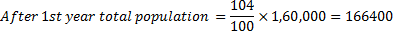In 2nd year , 5% increased

After 2 years the total population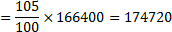In 3rd year, 8% increased

After 3 years the total population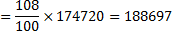3) Pankaj gave 50 percent of the amount to akash. Akash in turn gave two-fifth of the amount to venu. After paying a bill of 500 rupees, venu now have 8000 rupees left with him. Find the amount hold by pankaj initially.

a) 41500

b) 42500

c) 43500

d) 44500

e) none of these

b)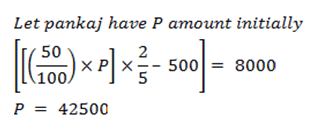4)  If A = x% of y and B = y% of x, then which of the following is true?

a) Relationship between A and B cannot be determined

b) A is smaller than B

c) None of these

d) If x is smaller than y, then A is greater than B

e) A is greater than B

c)

A=XY/100

B=XY/100

5) The cost of raw material of a product increases by Rs 30, the manufacturing cost increases by Rs 20 and the selling price of the product increases by Rs 60. The raw material and the manufacturing cost, originally, formed Rs 40 and Rs 60 of the total cost respectively. If the original profit was one-fourth the original manufacturing cost, find the new profit percentage approximately.

a) 17.23%

b) 15.48%

c) 16.67%

d) 14.38%

e) 18.66%

c)

manufacturing cost = Rs 60

Raw materials cost = Rs 40

Original Profit 1/4 of Manufacturing Cost = 15 rs

So original selling is price 60 + 40 + 15=  Rs 115

New raw material cost = 40 + 30 = 70

New manufacturing cost = 60 + 20 = 80

New cost of the product = 150

New selling price = 115 + 60 = 175

New profit percentage = (27/175)*100=16.666=16.7

6) If the price of petrol increases by 25% and Raj intends to spend only an additional 15% on petrol, by how much % will he reduce the quantity of petrol purchased?

a) 10%

b) 12%

c) 18%

d) 16%

e) 8%

e)

Let the price of 1 litre of petrol be Rs.x and let Raj initially buys ‘y’ litres of petrol.

Therefore, he would have spent Rs. xy on petrol.

When the price of petrol increases by 25%, the new price per litre of petrol is 1.25x.

Raj intends to increase the amount he spends on petrol by 15%.

i.e., he is willing to spend xy + 15% of xy = 1.15xy

Let the new quantity of petrol that he can get be ‘q’.

Then, 1.25x *q = 1.15xy

As the new quantity that he can buy is 0.92y, he gets 0.08y lesser than what he used to get earlier.

Or a reduction of 8%.

7) B as a percentage of A is equal to A as a percentage of (A+B) . Find B as a percentage of A.

a) 73%

b) 82%

c) 62%

d) 96%

e) 99%

c)

B/A= ( A/(A/B))

B/A=X

X=  1/(1+(B/A))

X=1/(1+X)

solving we get x=0.62

x=62%

8) In a competitive examination in State ‘A’, 6% candidates got selected from the total appeared candidates. State ‘B’ had an equal number of candidates appeared and 7% candidates got selected with 80 more candidates got selected than State ‘A’. What was the number of candidates appeared from each state?

a) 8000

b) 8400

c) 7600

e) None of these

a)

Let the number of candidates appearing from each state be X.

Then,

7% of X – 6% of X = 80

Or, 1% of X = 80

Or, X = 8*100

X = 8000.

9) A part of sum of Rs. 20,000 is lent at 10% and the remaining such at 12% per annum. If the average rate of interest is 10.2%, then the two parts are

a) 2000, 18000

b) 18000, 2000

c) 15000, 7000

d) 9000, 7000

e) None of these

b)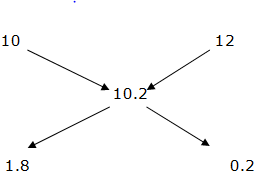First Part =(1.8/2)*20000

=0.9*20000=18000

Second Part = 20000-18000

Second Part = 2000

Second Part = 20000-18000

Second Part =2000

10) A shop keeper employed a servant at a monthly salary of Rs. 4000. In addition to it, he agreed to pay him a commission of 20% on the monthly sale. How much sale in rupees should the servant do if he wants monthly income as Rs. 8000?

a) 10,000

b) 20,000

c) 30,000

d) 40,000

e) None of these